# Thread

Programs run sequentially from top to bottom, left to right. If you run the code along with a pencil, it will look like a thread. So the program execution flow is called a thread. So far we have seen only single-threaded programs. In this article, I'll show you how to make a program that has more than two threads. Such a program is called a multi-thread program.

### How to create a multi-threaded program

1. Inherit Thread class
2. Implement the Runnable interface

## Comparison of single-threaded and multithreaded programs

### Single-threaded example

###### SingleThread.java
```package net.java_school.thread.test;

public class SingleThread {

public void doA() {
char[] alphabet = {'A','B','C','D','E','F','G','H','I','J'};
int length = alphabet.length;
for ( int i=0; i < length; i++ ) {
System.out.print(alphabet[i]);
}
}

public void doB() {
for (int i = 0; i < 10; i++) {
System.out.print(i);
}
}

public static void main(String[] args) {
SingleThread st = new SingleThread();
st.doA();
st.doB();
}

}
```

DoA() and doB() are executed on the same thread. Therefore doB() is executed after doA() ends.

### Multi-threaded example

Let's turn our example into a multi-threaded example.

#### Creating a multi-threaded program by inheriting the Thread class.

We will implement doA() and doB() to execute on different threads, respectively. First, I will demonstrate how to inherit a Thread. If you call the start() method after creating the object, another thread will be created and doA () will be executed on the new thread.

###### MultiThread1.java
```package net.java_school.thread.test;

public class MultiThread1 extends Thread {

public void doA() {
char[] alphabet = {'A','B','C','D','E','F','G','H','I','J'};
int length = alphabet.length;
for ( int i = 0; i < length; i++ ) {
System.out.print(alphabet[i]);
try {
//Take 0.01 seconds to allow another thread to take control.
Thread.sleep(10);
} catch (InterruptedException e) {
e.printStackTrace();
}
}
}

public void doB() {
for (int i = 0; i < 10; i++) {
System.out.print(i);
try {
//Take 0.01 seconds to allow another thread to take control.
Thread.sleep(10);
} catch (InterruptedException e) {
e.printStackTrace();
}
}
}

public void run() {
doA();
}

public static void main(String[] args) {
MultiThread1 mt1 = new MultiThread1();
mt1.start();
mt1.doB();
}
}
```

#### Create a multi-threaded program by implementing the Runnable interface.

The highlighted part of the main method is: Creates a MultiThread2 object. Call the Thread constructor and pass in a reference to the MultiThread2 object as an argument. The parameter type of this constructor is the Runnable interface.

###### MultiThread2.java
```package net.java_school.thread.test;

public class MultiThread2 implements Runnable {

public void doA() {
char[] alphabet = {'A','B','C','D','E','F','G','H','I','J'};
int length = alphabet.length;
for ( int i = 0; i < length; i++ ) {
System.out.print(alphabet[i]);

try {
//Take 0.01 seconds to allow another thread to take control.
Thread.sleep(10);
} catch (InterruptedException e) {
e.printStackTrace();
}
}

}

public void doB() {
for (int i = 0; i < 10; i++) {
System.out.print(i);

try {
//Take 0.01 seconds to allow another thread to take control.
Thread.sleep(10);
} catch (InterruptedException e) {
e.printStackTrace();
}
}
}

public void run() {
doA();
}

public static void main(String[] args) {
MultiThread2 mt2 = new MultiThread2();
Thread t = new Thread(mt2);
t.start();
mt2.doB();
}
}
```

### Critical Section

A critical section is a piece of code that accesses a shared resource. Let's look at an example of the problems that occur if the critical section is not handled properly. In the bank example, change the withdraw() method of NormalAccount as shown below. The reason for the change is to increase the probability that the balance of the normal account becomes negative.

```@Override
public void withdraw(long amount)  {
try {
Thread.sleep(5);
} catch (InterruptedException e) {
e.printStackTrace();
}

if (amount <= balance) {
Transaction transaction = new Transaction();
Calendar cal = Calendar.getInstance();
Date date = cal.getTime();
transaction.setTransactionDate(Account.DATE_FORMAT.format(date));
transaction.setTransactionTime(Account.TIME_FORMAT.format(date));
transaction.setAmount(amount);
transaction.setKind(Account.WITHDRAW);
balance = balance - amount;
transaction.setBalance(this.balance);
transactions.add(transaction);
} else {
throw new InsufficientBalanceException("There is not enough balance.");
}

}
```

Create a test class.

```package net.java_school.bank;

public class ThreadTest extends Thread {
private Bank bank = new ShinhanBank();
private Account account;

public ThreadTest() {
bank.addAccount("101", "Alison", Account.NORMAL);
account = bank.getAccount("101");
account.deposit(1000);
}

public void withdrawTest() {
int i = 0;
do {
i++;
try {
account.withdraw(100);
} catch (InsufficientBalanceException e) {}

try {
Thread.sleep(10);
} catch (InterruptedException e) {
e.printStackTrace();
}
} while (i < 100);

}

public void run() {
withdrawTest();
}

public static void main(String[] args){
ThreadTest t = new ThreadTest();
t.start();
t.withdrawTest();
Account account = t.bank.getAccount("101");
System.out.println(account);
}
}
```

When you test several times, there is a case where the balance of the Noraml Account is negative.

C:\ Command Prompt
```C:\java\Bank\bin>java net.java_school.bank.ThreadTest
101|Alison|0|Normal
C:\java\Bank\bin>java net.java_school.bank.ThreadTest
101|Alison|0|Normal
C:\java\Bank\bin>java net.java_school.bank.ThreadTest
101|Alison|0|Normal
C:\java\Bank\bin>java net.java_school.bank.ThreadTest
101|Alison|-100|Normal
```

The cause of this problem is that the thread proceeds as shown below.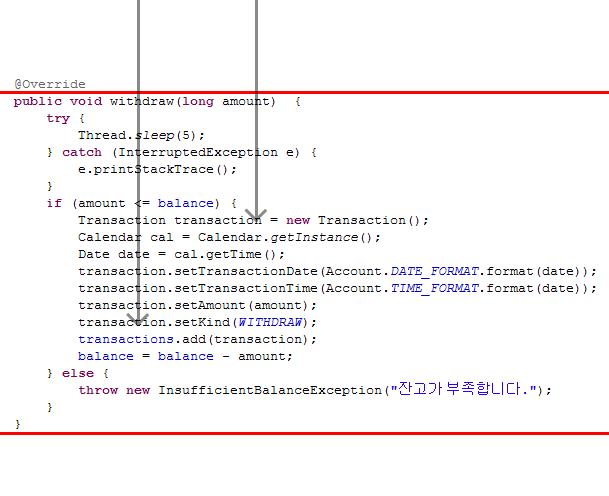To solve the problem, apply the synchronized keyword to the withdraw() method.
Applying synchronized to a method prevents other threads from entering until the thread entering the method exits the method.

```@Override
public synchronized void withdraw(long amount)  {
.. Same as existing code ..
}
```

Test it several times and make sure that there is no longer a negative balance in your normal account.
As shown in the figure below, the second thread will not be able to enter the method until the first thread has left the method.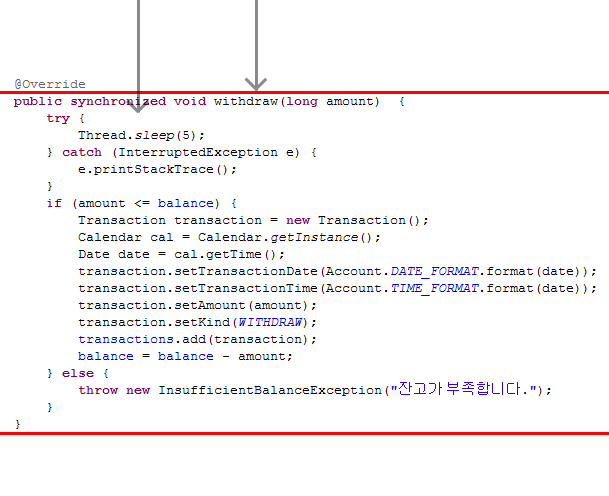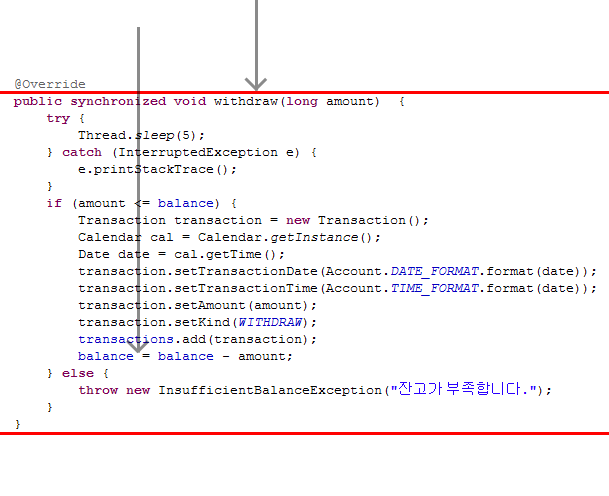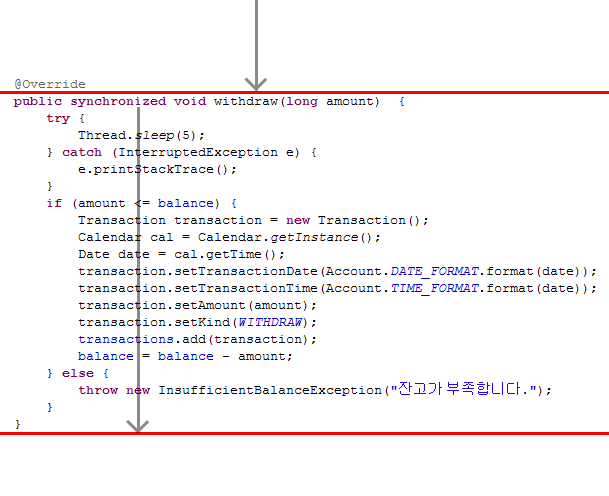When the first thread goes out of the method, the waiting thread enters the method.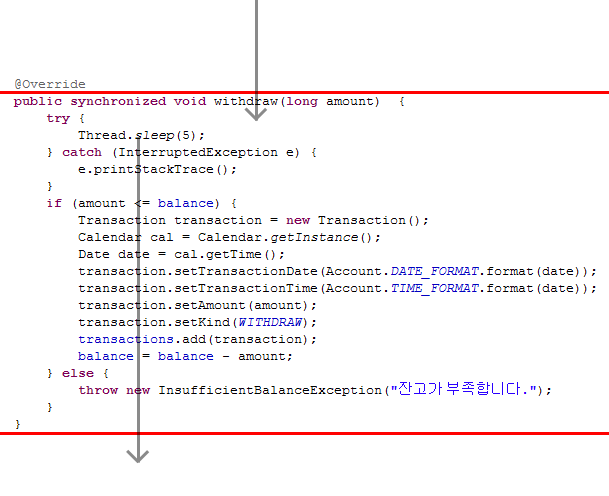Return the withdraw() method as shown below.

```@Override
public synchronized void withdraw(long amount)  {
if (amount > balance) {
throw new InsufficientBalanceException("There is not enough balance.");
}
balance = balance - amount;
Transaction transaction = new Transaction();
Calendar cal = Calendar.getInstance();
Date date = cal.getTime();
transaction.setTransactionDate(Account.DATE_FORMAT.format(date));
transaction.setTransactionTime(Account.TIME_FORMAT.format(date));
transaction.setAmount(amount);
transaction.setKind(Account.WITHDRAW);
transaction.setBalance(this.balance);
transactions.add(transaction);
}
```

Apply the synchronized keyword to the deposit() method.

```public synchronized void deposit(long amount) {
//.. Same as existing code ..
}
```

The Vector is thread-safe, but the ArrayList is not. All methods of Vector are synchronized.